#### Methods for Estimating the Standard Deviation

When control limits are computed from the input data, four methods are available for estimating the process standard deviation. Three methods (referred to as the default, MVLUE, and RMSDF) are available with subgrouped data. A fourth method is used if the data are individual measurements (see Default Method for Individual Measurements).

##### Default Method for Subgroup Samples

This method is the default for EWMA charts using subgrouped data. The default estimate ofis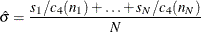where N is the number of subgroups for which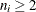,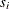is the sample standard deviation of the ith subgroup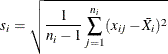and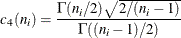Here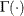denotes the gamma function, and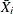denotes the ith subgroup mean. A subgroup standard deviationis included in the calculation only if. If the observations are normally distributed, then the expected value ofis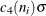. Thus,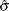is the unweighted average of N unbiased estimates of. This method is described in the American Society for Testing and Materials (1976).

##### MVLUE Method for Subgroup Samples

If you specify SMETHOD=MVLUE, a minimum variance linear unbiased estimate (MVLUE) is computed for. Refer to Burr (1969, 1976) and Nelson (1989, 1994). The MVLUE is a weighted average of N unbiased estimates ofof the form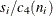, and it is computed as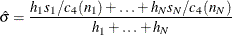where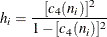A subgroup standard deviationis included in the calculation only if, and N is the number of subgroups for which. The MVLUE assigns greater weight to estimates offrom subgroups with larger sample sizes, and it is intended for situations where the subgroup sample sizes vary. If the subgroup sample sizes are constant, the MVLUE reduces to the default estimate.

##### RMSDF Method for Subgroup Samples

If you specify SMETHOD=RMSDF, a weighted root-mean-square estimate is computed foras follows: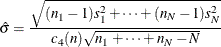The weights are the degrees of freedom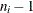. A subgroup standard deviationis included in the calculation only if, and N is the number of subgroups for which.

If the unknown standard deviationis constant across subgroups, the root-mean-square estimate is more efficient than the minimum variance linear unbiased estimate. However, in process control applications it is generally not assumed thatis constant, and ifvaries across subgroups, the root-mean-square estimate tends to be more inflated than the MVLUE.

##### Default Method for Individual Measurements

When each subgroup sample contains a single observation (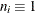), the process standard deviationis estimated as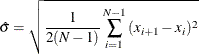where N is the number of observations, and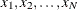are the individual measurements. This formula is given by Wetherill (1977), who states that the estimate of the variance is biased if the measurements are autocorrelated.